Courses

# Competition Level Test: Probability- 2

## 30 Questions MCQ Test Mathematics For JEE | Competition Level Test: Probability- 2

Description
This mock test of Competition Level Test: Probability- 2 for JEE helps you for every JEE entrance exam. This contains 30 Multiple Choice Questions for JEE Competition Level Test: Probability- 2 (mcq) to study with solutions a complete question bank. The solved questions answers in this Competition Level Test: Probability- 2 quiz give you a good mix of easy questions and tough questions. JEE students definitely take this Competition Level Test: Probability- 2 exercise for a better result in the exam. You can find other Competition Level Test: Probability- 2 extra questions, long questions & short questions for JEE on EduRev as well by searching above.
QUESTION: 1

### The equation of line of intersection of the planes x + 2y + z = 3 and 6x + 8y + 3z = 13 can be written as

Solution:

Let the d.r’s of a required line be a, b and c. Since, the normal to the given planes x + 2y + z = 3 and 6x + 8y + 3z = 13 are perpendicular to the line.
∴ a +2b+c = 0 and 6a + 8b + 3c = 0
⇒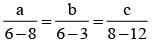⇒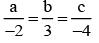or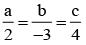Also, line passes through (2, -1, 3).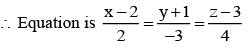QUESTION: 2

### The direction cosines of two lines are such that l + m + n = 0, l2 + m2 – n2 = 0, then angle between them is

Solution:

l + m + n = 0
and  l2 + m2 - n2 = 0
l2 + m2 (- l - m)2 = 0
2lm = 0  (l = 0 or m = 0)
If l = 0 then n = - m
l : m : n = 0 : l : -1
If m = 0 then n = - l
l : m : n = l : 0 : -1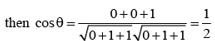⇒ θ = π/3

QUESTION: 3

### The point of intersection of the lines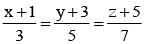and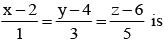Solution:

Find general pt. of both line and equate them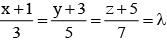⇒ (3λ - 1, 5λ - 3, 7λ - 5 ) .......(i)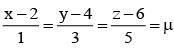⇒ (μ +2, 3μ + 4, 5μ + 6) .......(ii)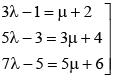on solving λ = 1/2,  & μ = -3/2
on putting λ = 1/2, & μ = -3/2 we get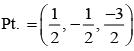QUESTION: 4

If the straight lines x = 1 + s, y = - 3 - λs, z = 1 + λs and x = t/2, y = 1 + t, z = -t + 2 with parameters s and t respectively are coplanar, then λ equals

Solution:

Given line can be rewritten as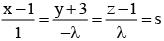and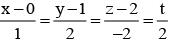since two line are coplanar, then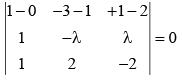λ = -2

QUESTION: 5

Shortest distance between the line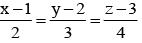and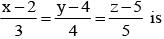Solution:

Given, lines can be written as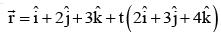and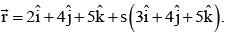∴ Requires SD =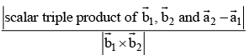Where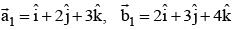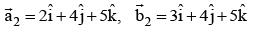∴ Requires SD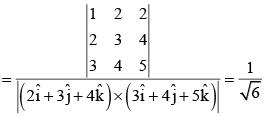QUESTION: 6

If P(B) = 3/4,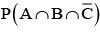= 1/3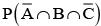= 1/3, then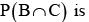Solution: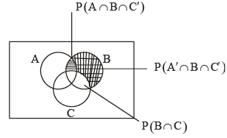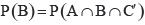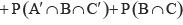QUESTION: 7

If events are independent and P(A) = 1/3, P(B) = 1/3, P(C) = 1/4 then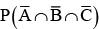is equal to

Solution: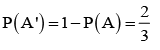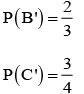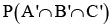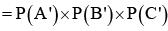QUESTION: 8

A fair die is tossed eight times. The probability that a third six is observed on the eight throw is

Solution:

Required probability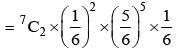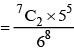QUESTION: 9

For k = 1, 2, 3, the box Bk contains k red balls and (k + 1) white balls. Let P(B1) = 1/2, P(B2) = 1/3, P(B3) = 1/6. A box is selected at random and a ball is drawn from it. If a red ball is drawn, then probability that it has come from box B2 is

Solution:

Use Baye’s Theorem formula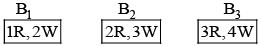A : Red ball is drawn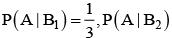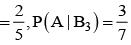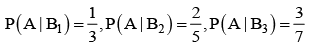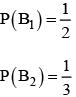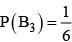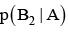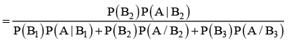= 14/39

QUESTION: 10

Let A and B be two event such that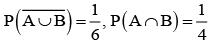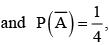stands for complement of event A. Then events A and B are

Solution: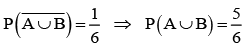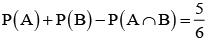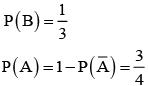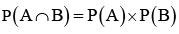Independent event but not equally likely

QUESTION: 11

Three of the six vertices of a regular hexagon are chosen at random. The probability that triangle with three chosen vertices is an equilateral triangle is

Solution:

Total No. of ways = 6C3
No. of favorable ways = 2

QUESTION: 12

If A and B are two events such that P(A∪B) = 3/4, P(A∩B) 1/4,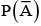= 2/3, then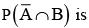Solution: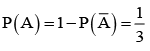P(A∪B) = P(A) = P(A) + P(B) - P(A∩B)
P(B) = 2/3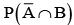= P(B) - P(A∩B) =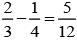QUESTION: 13

Three critics review a book. Odds in favour of book are 5 : 2, 4 : 3 and 3 : 4, respectively for the three critics. The probability that majority are in favour of the book is

Solution:

Probability that first critic favour is P(ε1) = 5/7
Similarly, P(ε2) = 4/7  P(ε3) = 3/7
Majority are in favour if at least two favour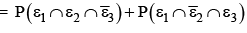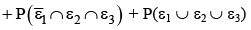QUESTION: 14

Out of a set of integers given by {1, 2, 3, …. 30}, three numbers are selected at random. Find the probability the sum of the number chosen is divisible by 3.

Solution:

number of type 3k → 10
3k + 1 → 10
3k + 2 → 10
Either all the no. are of the same type or one No. from each type

QUESTION: 15

A is a set containing n element. A subset P of A is chosen at random. The set A is reconstructed by replacing the element of P. A set ‘Q’ is again chosen at random. Find probability such that P∩Q = φ.

Solution:

For 1 element not to be present in P∩Q possibilities for n element ⇒ Total
No. of fovourable cases = 3n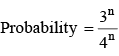QUESTION: 16

If the integers m and n are chosen at random from 1 to 100, then probability that a no. of form 7m + 7n is divisible by 5 equals

Solution:

Consider last digit of power of 7
last digit
74k → 1
74k+1 →​ 7
74K+2 →​ 9
74k+3 →​ 3
7m + 7n is divided by 5 if
(i) m → 4k + 1, n → 4k + 3
(ii) m→ 4k + 2 and n → 4k
(iii) m→ 4k+3 andn→ 4k+1
(iv) m → 4k and n → 4k + 2

QUESTION: 17

If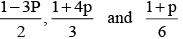are the probability of three mutually exclusive and exhaustive events, then the set of all value of p is

Solution:

Since events are mutually exclusive and exhaustive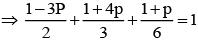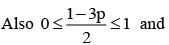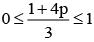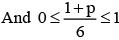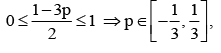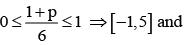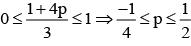Hence the set of value satisfying all the above inequalities are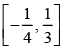QUESTION: 18

A man alternately tosses a coin and throws a dice beginning with the coin. The probability that he gets a head in the coin before he gets a 5 or 6 in the dice is

Solution:

Probability of getting head = 1/2 and probability of throwing 5 or 6 with a dice = 2/6 1/3 . He starts with a coin and alternately tosses the coin and throws the dice and he will if he get a head before he get 5 or 6.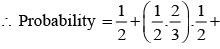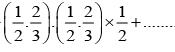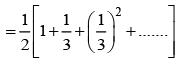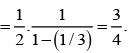QUESTION: 19

Three distinct numbers are selected from first 100 natural numbers. The probability that all the three numbers are divisible by 2 and 3 is

Solution:

The numbers should be divisible by 6. Thus, the number of favourable ways is 16C3 (as there are 16 numbers in first 100 natural numbers, divisible by 6).
Required probability isQUESTION: 20

A student appears for test I, II and III. The student is successful if he passes either in test I and II or test I and III. The probability of the student passing in test I, II, III are p, q and 1/2 respectively. If the probability that the student is successful is 1/2, then

Solution:

Let A, B and C be the events that the student is successful in test I, II and III respectively, then P(the student is successful)

P[A∩B∩C')∪(A∩B'∩C)∪(A∩B∩C)]
= P(A∩B∩C') + P(A∩B'∩C) + P(A∩B∩C)
P(A).P(B).P(C') + P(A)P(B')P(C) + P(A)P(B)P(C)
{∴ A, B, C are independent}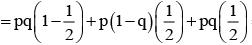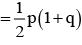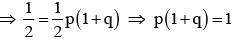This equation has infinitely many values of p and q.

QUESTION: 21

It is given that events A and B are such that P(A) = 1/4, P(A/B) = 1/2 and P(B/A) = 2/3, then P (B) is

Solution:

P(A/B) = P(A⋂B)/P(B)
P(B/A) = P(B⋂A)/P(A)
2/3 = P(B⋂A)/(1/4)
P(A⋂B) = 2/3*1/4
= 1/6
Therefore, P(A/B) = P(A⋂B)/P(B)
1/2 = 1/6P(B)
P(B) = 1/3

QUESTION: 22

Out of a set of integers given by {1, 2, 3, …. 30}, three numbers are selected at random. Find the probability the sum of the number chosen is divisible by 3.

Solution:

number of type 3k → 10
3k + 1 → 10
3k + 2 → 10
Either all the no. are of the same type or one No. from each type

QUESTION: 23

The equation of plane passing through the point (0, 7, -7) and containing the line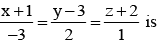Solution:

The equation of plane passing through (0, 7, -7) is a(x – 0) + b(y – 7) + c(z + 7) = 0
Plane contains line and passes through (-1, 3, -2)
∴ a(-1) + b(3 – 7) + c(-2 + 7) = 0
-3a + 2b + c = 0
∴ a : b : c = 1 : 1 : 1
x + y + z = 0

QUESTION: 24

The angle between line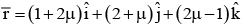and plane 3x – 2y + 6z = 0 is (μ is scalar)

Solution:

D. R’s of line are 2 : 1 : 2
D.R’s of Normal to plane is :3 : -2 : 6
Angle between line and plane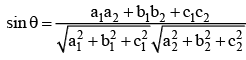QUESTION: 25

Let P(3, 2, 6) be a point in space and Q be a point on line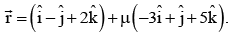Then value of m for which the vector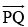is parallel to the plane x-4y + 3z = 1 is

Solution:= i(- 2 - 3μ) + j(μ - 3) + k(5μ - 4)is parallel to x - 4y + 3z = 1
1(- 2 - 3μ) + 4(μ - 3) + 3(5μ - 4) = 0
μ = 1/4

QUESTION: 26

The equation of the plane through the point (-1, 2, 0) and parallel to the line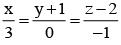and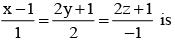Solution: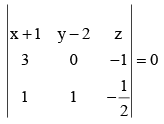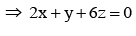QUESTION: 27

Let A (1,1,1) , B (2, 3, 5) and C (-1, 0, 2) be three points, then equation of a plane parallel to the plane ABC which is at a distance 2 from origin is

Solution: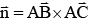A (1,1,1), B (2,3,5), C(-1,0,2) direction ratios of AB are < 1,2,4 >
Therefore, direction ratios of normal to plane ABC are < 2, -3,1 >
As a result, equation of the required plane is 2x – 3y +z = k then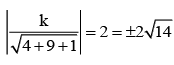Hence, equation of the required plane is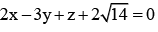QUESTION: 28

The distance between the line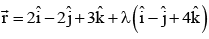and the plane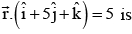Solution: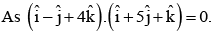Therefore, the line and the plane are parallel. A point on the line is (2, -2, 3). Required distance = distance of (2, -2, 3) from the given plane x + 5y + z - 5 = 0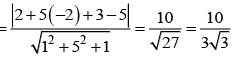QUESTION: 29

If the distance between the plane x - 2y + z = d and the plane containing the line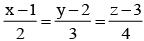and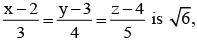then |d| is

Solution:

Equation of plane containing the given lines is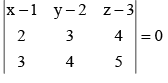⇒ (x - 1) (-1) - (y - 2) (-2) + (z - 3) (-1) = 0
⇒ -x  + 1 + 2y - 4 - z + 3
⇒ -x + 2y - z = 0
Given plane is
x - 2y + z = d .....(ii)
Eqs. (i) and (ii) are parallel
Now, distance between planes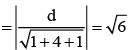⇒ |d| = 6

QUESTION: 30

A plane π passes through the point (1, 1, 1). If b, c, a are the direction ratios of a normal to the plane, where a, b, c (a < b < c) are the prime factors of 2001, then the equation of the plane π is

Solution:

2001 = 3 x 23 x 29 and (3+23+29)
= 55 ⇒ a = 3, b = 23, c = 29